# ISEE Middle Level Math : Distributive Property

## Example Questions

### Example Question #21 : Distributive Property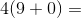Possible Answers: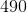Correct answer:Explanation:

First complete the operations within the parentheses: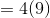Then, multiply: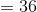Answer:### Example Question #22 : Distributive Property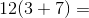Possible Answers: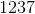Correct answer:Explanation:

First complete the operations in parentheses: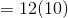Then, multiply: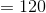Answer:### Example Question #23 : Distributive Property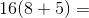Possible Answers: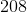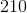Correct answer:Explanation:

First add the numbers in the parentheses. Then multiply: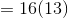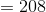Answer:### Example Question #24 : Distributive Property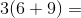Possible Answers: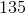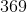Correct answer:Explanation:

Using the distibutive property, solve: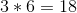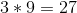Then, solve the equation: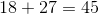Answer:### Example Question #25 : Distributive Property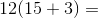Possible Answers: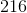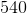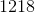Correct answer:Explanation:

Using the distibutive property, solve: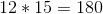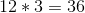Then, solve the equation: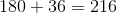Answer:### Example Question #591 : Numbers And Operations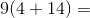Possible Answers: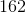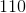Correct answer:Explanation:

Using the distibutive property, solve: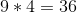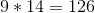Then, solve the equation: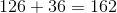Answer:### Example Question #592 : Numbers And Operations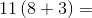Possible Answers: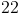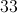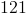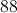Correct answer:Explanation:

First complete the addition in the parentheses, then multiply the two values:

11 (8 + 3)

= 11 (11)

= 121

### Example Question #593 : Numbers And Operations

Solve using the distributive property: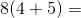Possible Answers: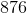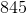Correct answer:Explanation:

First, multiply: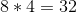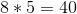Then, add: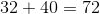Answer:.

### Example Question #594 : Numbers And Operations

Solve using the distributive property: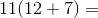Possible Answers: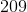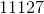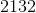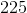Correct answer:Explanation:

First, multiply: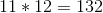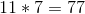Then, add: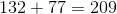Answer:.

### Example Question #595 : Numbers And Operations

Solve the equation.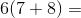Possible Answers: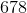Correct answer:Explanation:

Use the distributive property.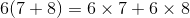Order of operations means that the multiplication must come first.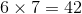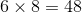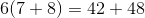Then, solve the equation: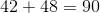Answer:### All ISEE Middle Level Math Resources